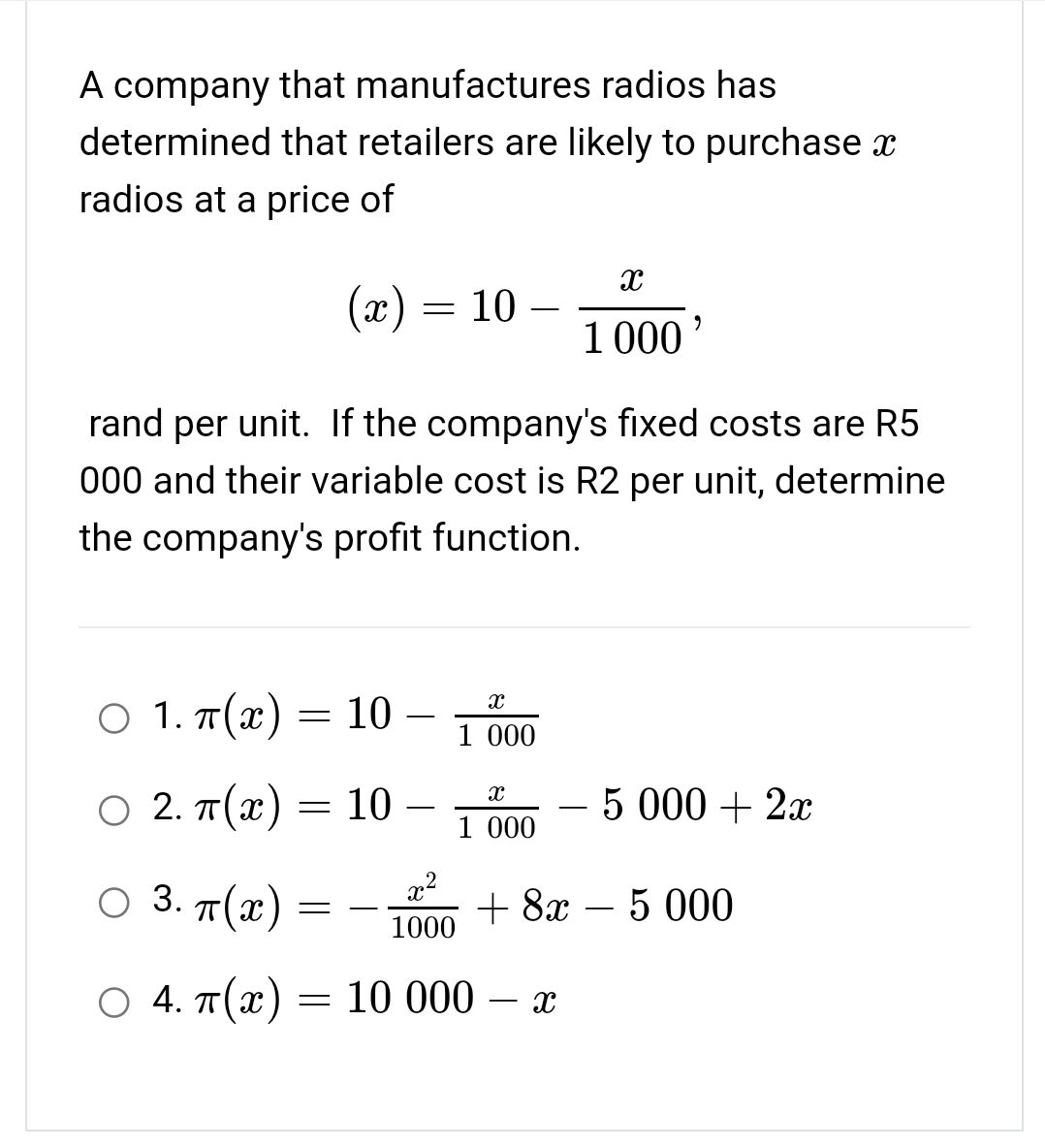### ¿Todavía tienes preguntas de matemáticas?

Pregunte a nuestros tutores expertos
Algebra
PreguntaA company that manufactures radios has determined that retailers are likely to purchase $$x$$ radios at a price of

$$( x ) = 10 - \frac { x } { 1000 } ,$$

rand per unit. If the company's fixed costs are $$R 5$$ $$000$$ and their variable cost is $$R 2$$ per unit, determine the company's profit function.

1. $$\pi ( x ) = 10 - \frac { x } { 1000 }$$

2. $$\pi ( x ) = 10 - \frac { x } { 1000 } - 5000 + 2 x$$

3. $$\pi ( x ) = - \frac { x ^ { 2 } } { 1000 } + 8 x - 5000$$

4. $$\pi ( x ) = 10000 - x$$